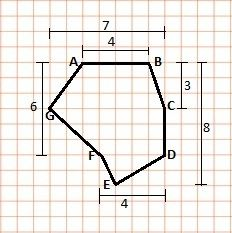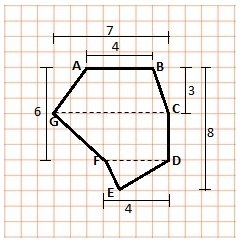# Worksheet on Area of a Polygon | Questions on Polygon Area | Area of a Polygon Worksheet

Worksheet on Area of a Polygon is helpful to the students who are willing to solve the questions on area of the pentagon, square, hexagon, octagon, and n-sided polygons. In case the students are preparing for any kind of test, then they can start preparation from this Area of the Polygon Worksheet. Utilize the Polygon Area Worksheets and score better grades in the exam.

In this Worksheet on Polygon Area, all types of questions are covered with hints. Learn How to Find the Area of a Polygon with the Problems available here. So, Practice problems from the Worksheet on Area of a Polygon as many times as possible so that you will understand the concept behind them.

1. Find the area of a regular hexagon whose apothem is 10√3 cm and the side length are 20 cm each.

Solution:

Given that,

Hexagon apothem = 10√3 cm

Side length of regular hexagon = 20 cm

Perimeter of the hexagon = (20 + 20 + 20 + 20 + 20 + 20)

= 120 cm

Area of a regular hexagon = ½ x perimeter x apothem

= ½ x 120 x 10√3

= 60 x 10√3 = 600√3

Therefore, area of a regular hexagon is 600√3 cm².

2. What is the area of a regular octagon with a side length of 12 m.

Solution:

Given that,

Side length of regular octagon = 12 m

Area of regular octagon = 2(1 + √2) a²

= 2(1 + √2) 12²

= 2(1 + √2) 144

= 288 (1 + √2) = 288(2.414)

= 695.293 cm²

Therefore, the area of a regular octagon is 695.293 cm².

3. Find the area of a regular pentagon of side:

(a) 5 cm

(b) 12 cm

Solution:

(a) 5 cm

Side of a regular pentagon a = 5 cm

Area of a regular pentagon = 1/4 ((√(5 (5 + 2 √5) a²))

= 1/4 ((√(5 (5 + 2 √5) 5²))

= 1/4 ((√(5 (5 + 2 √5) 25))

= 1/4 (6.88) (25)

= 172.04/4 = 43.01 cm²

Therefore, the pentagon area is 43.01 cm².

(b) 12 cm

Side of a regular pentagon a = 12 cm

Area of a regular pentagon = 1/4 ((√(5 (5 + 2 √5) a²))

= 1/4 ((√(5 (5 + 2 √5) 12²))

= 1/4 ((√(5 (5 + 2 √5) 144))

= (144/4) (6.88)

= 36 x 6.88 = 247.68 cm²

Therefore, area of a pentagon is 43.01 cm².

4. Find the area of a regular pentagon whose each side is 12 cm long and the radius of the inscribed circle is 6.5 cm.

Solution:

Given that,

The side length of pentagon = 12 cm

The radius of the inscribed circle = 6.5 cm

In ΔOMC, by Pythagoras theorem

OC² = OM² + MC²

6.5² = h² + 6²

42.25 = h² + 36

h² = 42.25 – 36

h² = 6.25

h = √(6.25)

= 2.5

The area of triangle ODC = 1/2 x DC x OM

= 1/2 x 12 x 2.5 = 6 x 2.5 = 15 cm²

The pentagon includes 5 triangles

So the area of the Pentagon is five times the area of the triangle

Pentagon area = 5 x area of one triangle

= 5 x 15 = 75 cm²

Therefore, the area of the pentagon is 75 cm².

5. Find the area of a regular pentagon whose each side is 5 cm long and the radius of the whose circumscribed circle is 3.5 cm.

Solution:

Given that,

Side length of regular pentagon a = 5 cm

The radius of circumscribed circle R = 3.5 cm

Area of the pentagon = n/2 × a × √(R² – a²/4)

= 5/2 x 5 x √(3.5² – 5²/4)

= 5/2 x 5 x √(12.25 – 25/4)

= 5/2 x 5 x √(12.25 – 6.25)

= 5/2 x 5 x √(6)

= 25/2 x 2.44

= 25 x 1.224 = 30.618 cm²

Therefore, the pentagon area is 30.618 cm².

6. What is the area of a parallelogram that has a base of 12 ¾ in and a height of 2 ½ in?

Solution:

Given that,

Base = 12 ¾ inches = 51/4 in

Height = 2 ½ inches = 5/2 in

Area of the parallelogram = base x height

= (51/4) x (5/2)

= 255/8 square inches.

Therefore, the parallelogram area is 255/8 sq inches.

7. The diagonals of a rhombus are 12 m and 26 m. What is the area of the rhombus?

Solution:

Given that,

Diagonals of a rhombus are a = 12 m, b = 26 m

Area of the rhombus = 1/2 (a x b)

= 1/2 (12 x 26) = 6 x 26 = 156 m²

Therefore, the area of rhombus is 156 m².

8. Find the area of polygon ABCDEFG.Solution:

The polygon can be split into two trapeziums and a triangle.The area of polygon ABCDEFG is given by the sum of the area of trapezium ABCG and CDFG and the area of triangle DEF.

Height of trapezium ABCG = 3 cm

Height of trapezium CDFG = (6 – 3) = 3 cm

Height of triangle DEF = (8 – 6) = 2 cm

Area of trapezium ABCG = (sum of parallel sides) × height/2

= (4 + 7)×3/2

= 33/2 = 16.5 cm²

Area of trapezium CDFG = (7 + 4) ×3/2

= 33/2 = 16.5 cm²

Area of triangle DEF = (base ×height)/2

= (4 × 2)/2 = 8/2

= 4 cm²

So, area of polygon ABCDEFG = area of ABCG + area of CDFG + area of DEF

= 16.5 + 16.5 + 4 = 37 cm²

9. Find the area of 15 sided polygon having a side length of 2 cm.

Solution:

Given that,

Number of sides of regular polygon = 15

Side length of polygon = 2 cm

Area of regular polygon A = [n * a² * cot(π/n)]/4

A = (15 * 2² * cot(π/15))/4

= (15 x 4 x 4.704)/4

= 282.24/4 = 70.56

Therefore, area of 15 sided polygon having 2 cm length is 70.56 cm².

10. Find the area, the perimeter of the regular heptagon with side length 15 cm and apothem is 25√3 cm.

Solution:

Given that,

The apothem of regular heptagon a = 25√3 cm

Side length = 15 cm

Perimeter of regular heptagon = 15 + 15 + 15 + 15 + 15 + 15 + 15

= 105 cm

The regular heptagon area = ½ x perimeter x apothem

= ½ x 105 x 25√3 = 2625√3/2 = 1312.5√3 cm²

Therefore, the perimeter and area of the heptagon is 105 cm, 1312.5√3 cm².

11. Find the regular hexagon area whose distance from the center of sides to the hexagon center is 32 cm.

Solution:

Given that,

The distance from the center of sides to the hexagon center = 32 cm

Regular hexagon area = (3√3)/2 × distance from the center of sides to the center of the hexagon

= (3√3)/2 x 32 = (3√3) x 16

= 48√3 cm²

Therefore, the hexagon area is 48√3 cm².